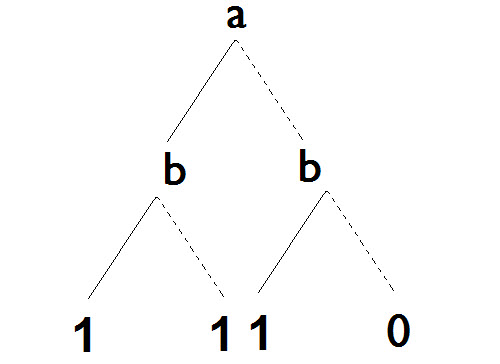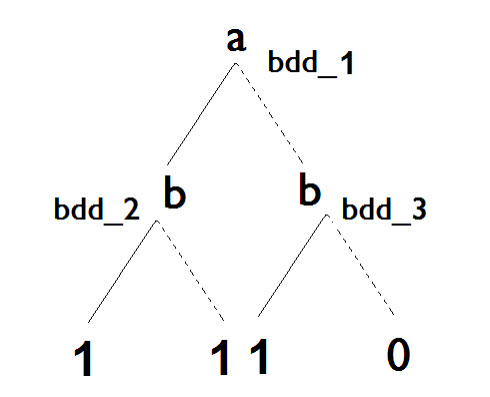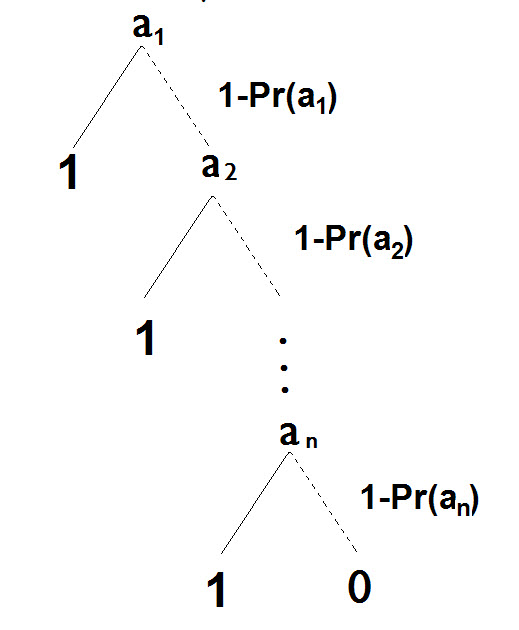# The Emperor’s New Truncation

## A Modern Fairy Tale

Once upon a time …

Many years ago there lived an Emperor who was so exceedingly fond of fine new clothes that he spent vast sums of money on dress. To him clothes meant more than anything else in the world. He took no interest in his army, nor did he care to go to the theatre, or to drive about in his state coach, unless it was to display his new clothes. He had different robes for every single hour of the day.

We all know how the story ends. The emperor, bare-ass naked, parades himself in front of his subjects. Only the voice of a little child declares that the emperor has no clothes.

And so it is now, with BDD. Everyone is so fond of new ideas, and they hope for new breakthroughs in PSA quantification, that some embrace every proposed innovation with “ooooos” and “ahhhhs”.

We, however, are like the child who asks himself, “But does he have anything on?

We all like fairy tales. But in this time of global need for renewable energy, it is up to each of us to insure proper and correct methods for nuclear PSA, not invisible cloth.

So forgive us if this modern fairy tale we will now tell causes us to dress in less than splendor, but assuredly covering our private parts.

Being too pedantic, the notions of truth tables, BDD, minterms, and minimal cut sets. For those with a more academic bent, please refer to the seminal paper, “Mathematical Foundations of Minimal Cut Sets” [Rauzy2000].

A truth table is a tabular way of representing a Boolean function. If we have a Boolean function `F(a,b) = a V b`, where “V” means OR, the truth table for the function would be:

a b a V b
True True True
True False True
False True False
False False False

Each row of the truth table is mutually exclusive, or disjoint (called a directed acyclic graph, DAG) of
a truth table, usually, we hope, a more compact representation, built top-down. So given the same function, `F(a,b) = a V b`, we might have this BDD structure:Think of the solid lines as assigning “true” to the variable from which they emanate, the dotted lines as assigning “false”, and the 1’s and 0’s indicating if the function is satisfied or not by the assignments. Each path in the graph is disjoint, like the rows of a truth table.

We can read the graph as indicating that if a and b are true, then the function is satisfied, if a is true, but b is false, the function is satisfied, and so a graph with a function called “if-thenelse”, usually written `ite(if, then, else) `, which means if a is true proceed down the left branch, if false, proceed down the right branch. So given the graph from the preceding page:Building the BDD top-down, we can represent it with this series of functions:
```BDD = bdd_1 bdd_1 = ite(a,bdd_2,bdd_3) bdd_2 = ite(b,1,1) bdd_3 = ite(b,1,0)```

Boolean function as an equation of variables “and”ed and then “or”ed together, the same as the disjoint paths of a BDD, or the rows of a truth table.

Using variable juxtaposition as “and”, “+” as “or”, and “~” as “not, we can create a minterm
representation of a Boolean function, `F(a,b,c) = ab + ~ac`. The set of minterms for F are
`MIN(F(a,b,c)) = abc + ab~c + ~abc + ~a~bc`.

These representations are logically equivalent, and if you build the truth table, you will see
that minterms are the “true” rows of a truth table.

a b c ab ac F
True True True True False True
True True False True False True
True False True False False False
True False False False False False
False True True False True True
False True False False False False
False False True False True True
False False False False False False

What is important to note here is that truth tables, BDDs, and minterms are mathematically equivalent representations of Boolean functions. Something cannot be true about one representation that is not true about the others.

Perhaps one representation can be computed faster than another, or understood easier than another, or a given property proven more simply than another, but we are up against a basic fact about mathematics and equivalent representations: if I can say it with a truth table, then I can say it about a BDD, and if I can’t, then I can’t.

[?] MCS, which are minterms without negated variables. So given the previous function, `F(a,b,c) = ab + ~ac = abc + ab~c + ~abc + ~a~bc`, the min cut sets of F are `MCS(F) = abc + ab + bc + c = ab + c`, which are the minterms, dropping negation.

The minterms of the min cut sets are `MIN(MCS(F)) = abc + ab~c + ~abc + ~a~bc + a~bc`. Notice that there is an additional term in green for `MIN(MCS(F))`, `a~bc`, which did not exist in the minterms.

Therefore the number of minterms in the min cut sets for a function F is always greater than, or equal to, the number of minterms of F, or mathematically, `Card(MIN(MCS(F))) >= Card(MIN(F))`. This is called the upper approximation, or monotone hull, of a Boolean function.

The point of the forgoing discussions was to show one very important idea: the disjoint terms of minterms, the disjoint paths of a BDD, and the disjoint rows of a truth table are all the same thing, with the disjoint terms of the minterms of min cut sets providing an upper approximation.

But why is any of this of interest to PSA?

Because we use these representations to quantify fault trees, which are a graphical representation of Boolean equations. And what we are interested in from the PSA point of view is the probability of the top event of a fault tree.

To calculate the probability of a Boolean equation in minterms or a BDD, it suffices to assign probabilities to each variable, substitute multiplication for “and”, addition for “or”, and subtract the probability for a variable from 1 for negation. Again using F

`F(a,b,c) = ab + ~ac`

If `Pr(a) = Pr(b) = Pr(c) = .1`, then for `MIN(F) = abc + ab~c + ~abc + ~a~bc`, the `Pr(F) = 1e-3 + 9e-3 + 9e-3 + 8.1e-2 = 1e-1`.

And for the minterms of the min cut sets, `MIN(MCS(F)) = abc + ab~c + ~abc + ~a~bc + a~bc = 1e-3 + 9e-3 + 9e-3 + 8.1e-2 + 9.e = 1.09e-1`, the upper bound approximation.

Notice that if we apply the same trick directly to `F(a,b,c) = ab + ~ac`, and convert it to min cut sets, `MCS(F) = ab + c`, we have the rare event approximation:

If this were the whole story, then we know, with proofs in hand, that we can calcultate the top event probabilities in PSA accurately.

But ain’t life grand? In the actual fault trees used in PSA it is impossible, in the most important cases, to completely construct truth tables, minterms, min cut sets, or BDDs.

So we rely on truncation. We say we are only interested in values for minterms or min cut sets or (now) BDD paths which are greater than a certain value, the truncation cutoff, C.

Now assume that you are interested only in the MCS whose probability is greater that a given cutoff C. Then you can remove all the minterms whose MCS probability is lower than C, where the MCS probability (called MCSPr) of a minterm (or of a product in general) is defined as the product of positive literal probabilities:

```Pr(~abc) = (1-Pr(a)) * Pr(b) * Pr(c) MCSPr(~abc) = Pr(b) * Pr(c)```

Let us denote by F/C the restriction of F to the minterms whose MCS probability is bigger than C and let MCS(F)/C be the set of MCS of F whose probability is bigger than c. Then the following theorem holds [Rauzy2000]:

`MCS(F/C) = MCS(F)/C`

Assume again that `Pr(a) = Pr(b) = Pr(c) = 0.1 and that C = 0.05`. Then we have:
`MCS(F/C(a,b,c)) = abc + ab~c + ~abc + ~a~bc` (minterms whose MCS probability is lower than C are in red).

Therefore:
```MCS(F/C) = MCS(~a~bc ) = { c } = MCS(F)/C (= { ab, c } )```

Note by the way that
`MIN(MCS(F/C)) = MIN(c) = abc + a~bc + abc + ~a~b~c`, and in therefore:
`MIN(MCS(F/C)) = MIN(F) U {A.-B.C} / {A.B.-C}` (where U stands for the union and / stands for the set difference)

So, what you get with truncated minterms is neither an upperapproximation (for you remove `ab~c`), nor a under-approximation (for you add a~bc), but an approximation of unknown error.

In other words, truncating minterms, truth tables or BDDs works but only from an MCS point of view, in other words, only if we include no negation.

Now the question is does probability truncation work with BDDs?

Assume that you truncate by considering the probability of branches as you build the BDD. Then you go nowhere, due to the specific structure of BDDs. Look at the function F:

`F = a1 + a2 + … an`

``` BDD(F) = bdd_1 bdd_1 = ite(a1,1, bdd_2) bdd_2 = ite(a2,1, bdd_3) bdd_n = ite(an,1,0) ```

Thus by eliminating all branches whose probability is below the cutoff C. Then as we build this BDD,[…] and we truncate counting the probability of 0-branches, we may reach a point where `(1-Pr(a1))*…*(1-Pr(ai)) < C`, therefore eliminating some perfectly valid MCS, and in doing so, under estimate the probability of the function F.complex function, we may add some perfectly invalid MCS, for example, let H, F, and G be Boolean functions such that:

``` H = ~FG F = a1 + … + an G = anB ```

The truncation of F may eliminate the MCS `{anb}`, which is part of the function G, and we
end up with an invalid MCS, anB, giving us an over estimation.

Together, BDD with truncation gives us an approximation of unknown error.

Notice that this is the same result as minterm truncation, which is what we would expect since they are equivalent representations. Moreover, this is the same objection which was raised concerning the Destructive Truth Table Method and Direct Probability Calculation.

No Free Lunch

As we said in CWTPRA, the rare event is fine for what it does

Proposed method is supposed to help with negation, but this is where it fails (success branches, delete-terms, recovery actions)

No mention in other papers of the limitations of the methods

No mention of previous work

What about keeping track of what is trunacted no mention of modules or variable ordering

Takes away from the real focus which is model clarity and transportability etc.

## Happily ever after?

But among the crowds a little child suddenly gasped out, “But he hasn’t got anything on.” And the people began to whisper to one another what the child had said. “He hasn’t got anything on.” “There’s a little child saying he hasn’t got anything on.” Till everyone was saying, “But he hasn’t got anything on.” The Emperor himself had the uncomfortable feeling that what they were whispering was only too true.